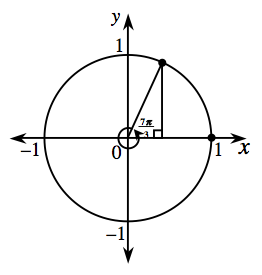### Home > CCA2 > Chapter 7 > Lesson 7.1.7 > Problem7-104

7-104.

What central angle, measured in degrees, corresponds to a distance around the unit circle of $\frac { 7 \pi } { 3 }$?

What number of degrees is equivalent to $\frac{\pi}{3}$ radians?

If you can't remember, calculate.

$\pi=180^{\circ}\ \ \ \ \ \ \ \ \frac{\pi}{3}=60^{\circ}$

Now multiply the angle by $7$.

$420^\circ$

1. What other angles will take you to the same point on the circle?

The distance around the unit circle is $2\pi$, no matter what point you start from.

$\frac{\pi}{3} \:\pm\:2\pi n$

2. Make a sketch of the unit circle showing the resulting right triangle.

The angle you are working with, $420^\circ$, is more than $360^\circ$. How much more?3. Find $\sin(\frac{7\pi}{3})$, $\cos(\frac{7\pi}{3})$ and $\tan(\frac{7\pi}{3})$ exactly.

Notice that the triangle formed is a $30^\circ-60^\circ-90^\circ$ triangle.

$\sin\left(\frac{7\pi}{3}\right)=\frac{\sqrt{3}}{2}$

$\cos\left(\frac{7\pi}{3}\right)=\frac{1}{2}$

$\tan\left(\frac{7\pi}{3}\right)=\sqrt{3}$